﻿ 基于特征熵权的集群分类算法 Cluster Classification Algorithm Based on Feature Entropy Weight

Computer Science and Application
Vol.08 No.05(2018), Article ID:24761,9 pages
10.12677/CSA.2018.85065

Cluster Classification Algorithm Based on Feature Entropy Weight

Dong Chen1, Yongbin Yu1, Chenxi Yang1, Yindong Chen1, Nyima Tashi2

1School of Information and Software Engineering, University of Electronic Science and Technology of China, Chengdu Sichuan

2College of Information and Technology, Tibet University, Lhasa TibetReceived: Apr. 12th, 2018; accepted: Apr. 26th, 2018; published: May 3rd, 2018ABSTRACT

Classification is a significant method in data mining. Most existing classification algorithms fail to make full use of the distribution information of various types of data. This paper proposes a cluster classification algorithm based on feature entropy weight. During the training, the information entropy weights are used to represent the data distribution of different categories and characteristics, and feature entropy weight vectors which can represent different data clusters can be obtained. During the test, if the test set is not divided into clusters of different types, it will be clustered according to the number of training clusters first. Then the feature entropy weight vectors of the test clusters are calculated, and the categories to which the test clusters belong are found by the cosine similarity. The experimental results show that the algorithm has high classification accuracy for data sets with distinct differences in the distribution of different types of features, and is more insensitive to abnormal data than existing classification algorithms. It can solve the problem that man-made labels are prone to errors to certain extent.

Keywords:Entropy Weight, Feature Distribution, Cluster, Classifier

1电子科技大学信息与软件工程学院，四川 成都

2西藏大学，信息科学与技术学院，西藏 拉萨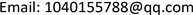1. 引言

2. 基于信息熵的特征分布向量表示

2.1. 信息熵

$H\left(X\right)=-\underset{i=1}{\overset{n}{\sum }}p\left({a}_{i}\right){\mathrm{log}}_{2}p\left({a}_{i}\right)$ (1)

2.2. 熵权与特征分布

$f\left({X}_{i}\right)=\frac{1}{\sqrt{\text{2π}}{\delta }_{i}}{\text{e}}^{-\frac{{\left({X}_{i}-{\mu }_{i}\right)}^{2}}{2{\delta }_{i}^{2}}}$ (2)

$H\left({X}_{i}\right)=-\frac{1}{\mathrm{ln}\left(n\right)}\underset{j=1}{\overset{n}{\sum }}{p}_{ji}\mathrm{ln}\left({p}_{ji}\right)$ (3)

${p}_{ij}=0$ ，则有： $\underset{p\to 0}{\mathrm{lim}}{p}_{ji}\mathrm{ln}\left({p}_{ji}\right)=0$

${W}_{i}=\frac{1-H\left({x}_{i}\right)}{k-\underset{i=1}{\overset{k}{\sum }}H\left({x}_{i}\right)}$ (4)

${A}_{l}=\left\{{W}_{1},{W}_{2},\cdots ,{W}_{k}\right\}$ (5)

2.3. 基于熵权的特征分布合理性仿真实验

$\left\{{X}_{1},{X}_{2}\right\}$ 为同一类样本的特征集合，且 $\left\{{X}_{1},{X}_{2}\right\}$ 服从高斯分布(2)。

${\mu }_{\text{1}}={\mu }_{\text{2}}=\text{50,}\text{\hspace{0.17em}}{\delta }_{\text{1}}={\delta }_{\text{2}}=\text{15}$ ，生成样点数num = 4000。采用控制变量法，分别研究三种参数变化对熵权变化影响情况。

2.3.1. 位置参数μi对权重分布的影响

${\mu }_{\text{2}}={\mu }_{\text{1}}+v,\text{\hspace{0.17em}}{\mu }_{\text{1}}=\text{50}$ ，v从0~100进行迭代， $\delta =\text{15}$ 固定不变，按2.2中过程生成特征熵权，如图1

2.3.2. 范围参数δ对权重分布的影响

${\delta }_{\text{2}}={\delta }_{\text{1}}+v,\text{\hspace{0.17em}}{\delta }_{\text{1}}=\text{1}$ ，v从0~100进行迭代， $\mu =\text{80}$ ，按2.2中过程生成特征熵权，如图2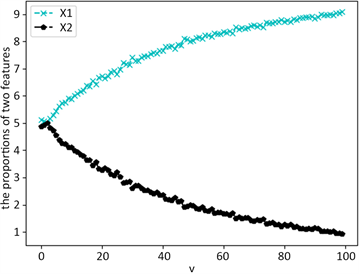Figure 1. Weight changes with location parameters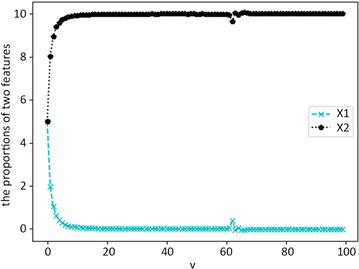Figure 2. Weight changes with range parameters

2.3.3. 范围参数δ对权重分布的影响

3. 集群分类器

3.1. 高斯混合聚类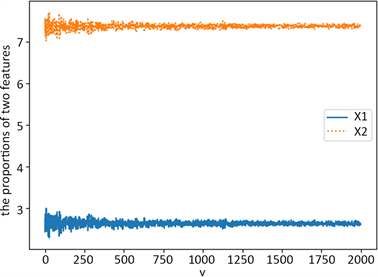Figure 3. Weight changes with the number of samples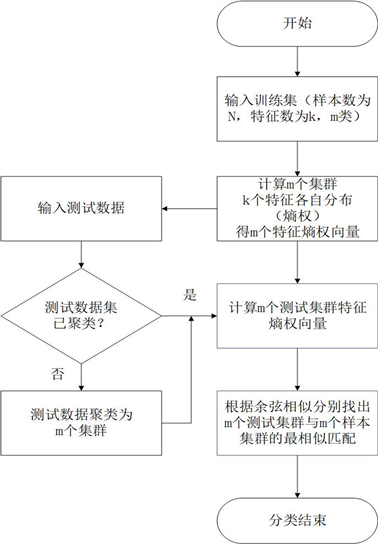Figure 4. The Algorithm flow of cluster Classification Algorithm Based on Feature Entropy Weight

$p\left(x\text{|}\theta \right)=\underset{l=1}{\overset{m}{\sum }}{\alpha }_{k}Ν\left(x|{\theta }_{l}\right)$ (6)

$Ν\left(x|{\theta }_{l}\right)=\frac{\text{1}}{\sqrt{\text{2π}}{\sigma }_{l}}{\text{e}}^{-\frac{{\left(x-{\mu }_{l}\right)}^{2}}{2{\delta }_{l}^{2}}}$ (7)

3.2 集群间的余弦相似匹配

$A=\left[\begin{array}{ccc}{w}_{11}^{A}& \cdots & {w}_{1k}^{A}\\ ⋮& \ddots & ⋮\\ {w}_{m1}^{A}& \cdots & {w}_{mk}^{A}\end{array}\right]=\left[\begin{array}{c}{A}_{1}\\ ⋮\\ {A}_{m}\end{array}\right]$ (8)

$a=\left[\begin{array}{c}{a}_{1}\\ ⋮\\ {a}_{m}\end{array}\right]=\left[\begin{array}{c}|{A}_{1}|\\ ⋮\\ |{A}_{m}|\end{array}\right]$ (9)

$B=\left[\begin{array}{ccc}{w}_{11}^{B}& \cdots & {w}_{1k}^{B}\\ ⋮& \ddots & ⋮\\ {w}_{m1}^{B}& \cdots & {w}_{mk}^{B}\end{array}\right]=\left[\begin{array}{c}{B}_{1}\\ ⋮\\ {B}_{m}\end{array}\right]$ (10)

$b=\left[\begin{array}{c}{b}_{1}\\ ⋮\\ {b}_{m}\end{array}\right]=\left[\begin{array}{c}|{B}_{1}|\\ ⋮\\ |{B}_{m}|\end{array}\right]$ (11)

$co{s}_{cd}=\frac{\underset{q=1}{\overset{k}{\sum }}{w}_{cq}^{A}{w}_{dq}^{B}}{\sqrt{\underset{q=1}{\overset{k}{\sum }}{\left({w}_{cq}^{A}\right)}^{2}}\sqrt{\underset{q=1}{\overset{k}{\sum }}{\left({w}_{dq}^{B}\right)}^{2}}}=\frac{{A}_{c}{B}_{d}^{\text{T}}}{{a}_{c}{b}_{d}}$ (12)

$cos=\frac{A{B}^{\text{T}}}{a{b}^{\text{T}}}$ (13)

3.3. 算法步骤

Step 1：将数据分为训练集与测试集，假设数据类别数为m，特征数为k。训练集中根据标签，将不同类别的样本划分为m个集群；

Step 2：根据 $H\left({X}_{i}\right)=-\frac{1}{\mathrm{ln}\left(n\right)}\underset{j=1}{\overset{n}{\sum }}{p}_{ji}\mathrm{ln}\left({p}_{ji}\right)$ 计算m个集群k个特征的信息熵。对m个集群求各特

Step 3：输入测试数据，并判断测试数据是否已经分成不同集群，而只需要判断集群所属类别，若是转Step 5；若否，则转Step 4；

Step 4：使用聚类算法，将测试数据聚成m个集群。

Step 5：根据熵权计算测试数据m个集群k个特征各自分布，得m个特征熵权向量 ${B}_{l}=\left\{{W}_{\text{1}}^{B},{W}_{2}^{B},\cdots ,{W}_{k}^{B}\right\}$ 。并以行为集群，列为特征，由 ${B}_{l}$ 组合成特征熵权矩阵 ${B}_{m×k}$ ，并计算每个集群特征熵权向量的模，放入特征熵权向量的模向量 ${b}_{m×1}$

Step 6：由 $cos=\frac{A{B}^{\text{T}}}{a{b}^{\text{T}}}$ 得训练集群与测试集群的余弦相关矩阵。找出每一列中余弦相似的最大值，即得该列所代表测试集群对应的训练集群类别。

4. 仿真结果及分析

4.1. 实验设计及参数设置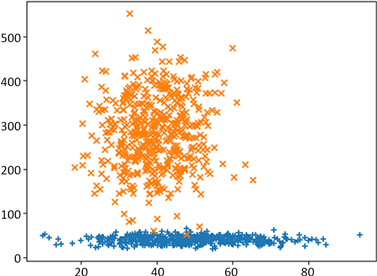Figure 5. Two types of training set data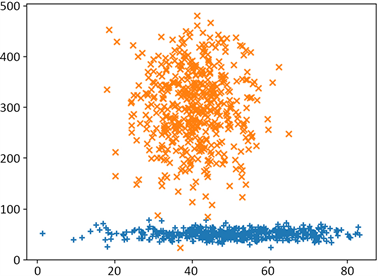Figure 6. Two types of test set data

4.2. 实验结果与分析

5. 结束语

Cluster Classification Algorithm Based on Feature Entropy Weight[J]. 计算机科学与应用, 2018, 08(05): 573-581. https://doi.org/10.12677/CSA.2018.85065

1. 1. Simic, S. (2009) Jensen’s Inequality and New Entropy Bounds. Applied Mathematics Letters, 22, 1262-1265. https://doi.org/10.1016/j.aml.2009.01.040

2. 2. Zheng, K.F. and Wang, X.J. (2018) Feature Selection Method with Joint Maximal Information Entropy between Features and Class. Pattern Recognition, 77, 20-29. https://doi.org/10.1016/j.patcog.2017.12.008

3. 3. 陈运, 周亮, 陈新, 陈建伟. 信息论与编码[M]. 北京: 电子工业出版社, 2015: 13-19.

4. 4. 罗可, 林睦纲, 郗东妹. 数据挖掘中分类算法综述[J]. 计算机工程, 2005, 31(1).

5. 5. Quinlan, J.R. (1979) Discovering Rules by Induction from Large Collections of Examples. In: Expert System in the Micro Electronic Age, 26-37.

6. 6. Trendowciz, A. and Jeffery, R. (2014) Classification and Regression Trees. Wiley Interdisciplinary Reviews Data Mining & Knowledge Discovery, 1, 14-23.

7. 7. Quinlan, J.R. (1993) C4.5:Programs for Machine Leaning. Morgan Kauffman, 23-30.

8. 8. Flach, P.A. and Lachiche, N. (2004) Naive Bayesian Classification of Structured Data. Machine Learning, 57, 233-269. https://doi.org/10.1023/B:MACH.0000039778.69032.ab

9. 9. Hsieh, H.Y. and Chen, N. (2012) Recognising Daytime and Nighttime Driving Images Using Bayes Classifier. IET Intelligent Transport Systems, 6, 482-493. https://doi.org/10.1049/iet-its.2010.0153

10. 10. Feng, X.D., Li, S.C., Yuan, C., Zeng, P. and Sun, Y. (2018) Prediction of Slope Stability Using Naive Bayes Classifier. KSCE Journal of Civil Engineering, 22, 941-950. https://doi.org/10.1007/s12205-018-1337-3

11. 11. Zhang, N.N., Wu, L.F., Yang, J. and Guan, Y. (2018) Naive Bayes Bearing Fault Diagnosis Based on Enhanced Independence of Data. Sensors (Basel, Switzerland), 18.

12. 12. Zhang, M.L. and Zhou, Z.H. (2007) ML-KNN: A Lazy Learning Approach to Multi-Label Leaming. Pattern Recognition, 40, 2038-2048. https://doi.org/10.1016/j.patcog.2006.12.019

13. 13. Peterson, L. (2009) K-Nearest Neighbor. Scholarpedia, 4, 156. https://doi.org/10.4249/scholarpedia.1883

14. 14. Denoeux, T. (2008) A k-Nearest Neighbor Classification Rule Based on Dempster-Shafer Theory. IEEE Transactions on Systems Man & Cybernetics, 25, 804-813. https://doi.org/10.1109/21.376493

15. 15. Lemeshow, S. (2004) Applied Logistic Regression. Journal of the American Sta-tistical Association, 85, 121-136.

16. 16. Keating, K.A. and Cherry, S. (2009) Use and Interpretation of Logistic Regression in Habitat-Selection Studies. Journal of Wildlife Management, 68, 774-789. https://doi.org/10.2193/0022-541X(2004)068[0774:UAIOLR]2.0.CO;2

17. 17. Wooff, D. (2004) Logistic Regression: A Self-Learning Text, 2nd. Journal of the Royal Statistical Society: Series A (Statistics in Society), 91, 433. https://doi.org/10.1111/j.1467-985X.2004.298_12.x

18. 18. Hong, S., You, T., Kwak, S., et al. (2015) Online Tracking by Learning Discriminative Saliency Map with Convolutional Neural Network. International Conference on Machine Learning, Lille, 6-11 July 2015, 597-606.

19. 19. Zhang, M.M., Li, W. and Du, Q. (2018) Diverse Region-Based CNN for Hyperspectral Image Classification. IEEE Transactions on Image Processing, 27, 2623-2634. https://doi.org/10.1109/TIP.2018.2809606

20. 20. Wang, J.Y. and Zhang, C. (2018) Software Reliability Prediction using a Deep Learning Model Based on the RNN Encoder-Decoder. Reliability Engineering & System Safety, 170, 73-82. https://doi.org/10.1016/j.ress.2017.10.019

21. 21. 杜景林, 严蔚岚. 基于距离权值的C4.5组合决策树算法[J]. 计算机工程与设计, 2018(1): 96-102.

22. 22. 童先群, 周忠眉. 基于属性值信息熵的KNN改进算法[J]. 计算机工程与应用, 2010, 46(3): 115-117.

23. 23. Shannon, C.E. (1948) A Mathematical Theory of Communication. Bell System Technical Journal, 27, 379-423. https://doi.org/10.1002/j.1538-7305.1948.tb01338.x

24. 24. Wang, Z. and Zhan, W. (2012) Dynamic Engineering Mul-ti-Criteria Decision Making Model Optimized by Entropy Weight for Evaluating Bid. Systems Engineering Procedia, 5, 49-54. https://doi.org/10.1016/j.sepro.2012.04.008